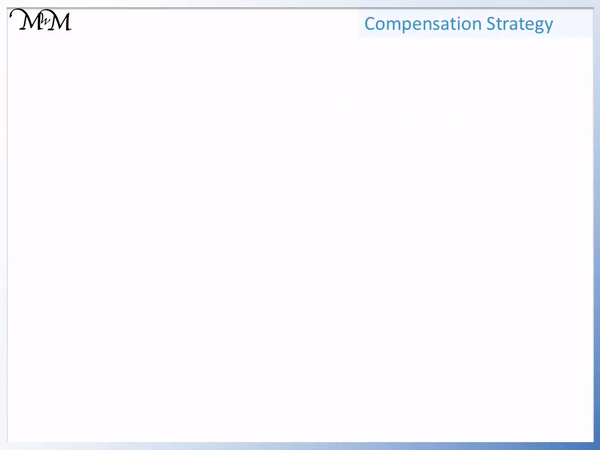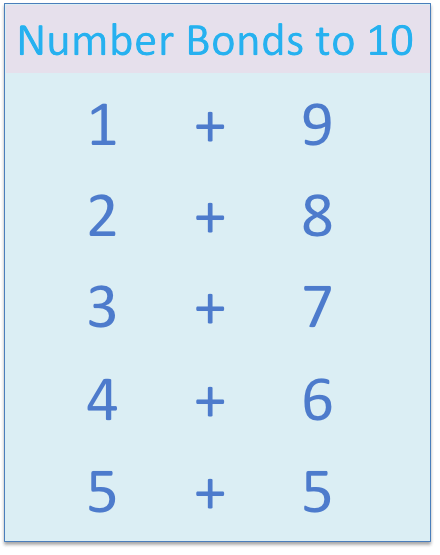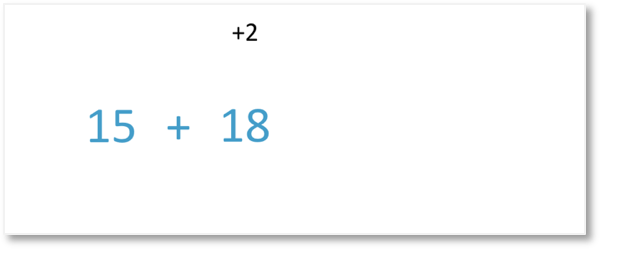# Addition using the Compensation Strategy• We want to add 7 + 8.
• Instead of 7 + 8, we will work out 7 + 10.
• 7 + 10 = 17.
• From our , 8 is two less than 10.
• Since 8 is two less than 10, we need to subtract 2 from 17.
• 7 + 8 = 15.

Adding ten and then subtracting two is easier than trying to add 8.• We want to find 15 + 18.
• It is easier to add 20 to 15 than it is to add 18.
• 15 + 20 = 35.
• 20 is two more than 18 so we have added 2 more than we needed to.
• We subtract two to compensate. So 35 – 2 = 33.
• 15 + 18 = 33.Supporting Lessons# What is the Addition Strategy for Compensation in Maths?

The compensation method is an addition strategy in which we add a larger number than we are required to add and then we subtract the extra amount afterwards.

We will look at addition examples in which we add numbers in the ten times table first. We will start by using our

to help us.We can instead work out 6 + 10, which equals 16.

This was an easier addition since the units remain as six, we just add an extra ten in front.

Looking at our number bonds to ten, we can see that 10 is three larger than 7.

We have added three too many.

We will subtract 3 from 16 to get our final answer.16 – 3 = 13

Therefore 6 + 7 = 13.

This method of adding more than we need and then subtracting the difference at the end is known as the addition compensation strategy.

Now that we have understood how to use the compensation method with our number bonds to ten, we will extend the strategy to look at adding larger numbers.

For example, in the addition example below, we have 15 + 18.To make this addition calculation easier we can add 2 to 18 to make our next multiple of ten, which is 20.15 + 20 = 35

Because we added 2 at the beginning, we now need to subtract 2 from our answer to compensate.35 – 2 = 33

Therefore,

15 + 18 = 33

With practice, the compensation method can be used as a mental addition strategy to quickly add 2-digit numbers.Now try our lesson on Mental Addition of 2-Digit Numbers where we learn how to quickly add 2-digit numbers in our head.

error: Content is protected !!